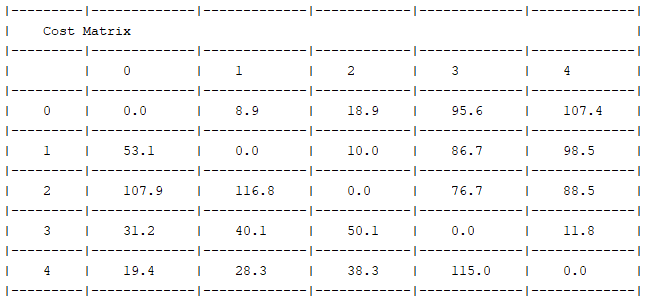# Pickup\Delivery does not works as expected

Hi,

I have very simple example and don’t understand why my example does not works as expected.
I have 1 vehicle, 2 shipments and cost time matrix.
The problem

The solution

I’m new in jsprit and fall with very simple example. What is wrong?
The expected solution is 0->1->2>3>4, but I have 0->3->4>1->2

The cost matrixThe code:

I would set cost for distance and time for the vehicle.

In the VehicleTypeImpl class, you can find the following:

``````    /**
* default cost values for default vehicle type
*/
private double fixedCost = 0.0;
private double perDistance = 1.0;
private double perTime = 0.0;
private double perWaitingTime = 0.0;
private double perServiceTime = 0.0;
``````

In your code, you did not set time cost for the vehicle type, and in the cost matrix you add time only and no distance, thus your route will always get 0 cost and will not be as expected. So you’d either set time cost for the vehicle type, or add distance to the cost matrix.

When I’ve added the cost per time it’s works as expected. Thanks a lot.
Is there an option to trace the jsrpit logic, that will help to understand what is happen under the hood?